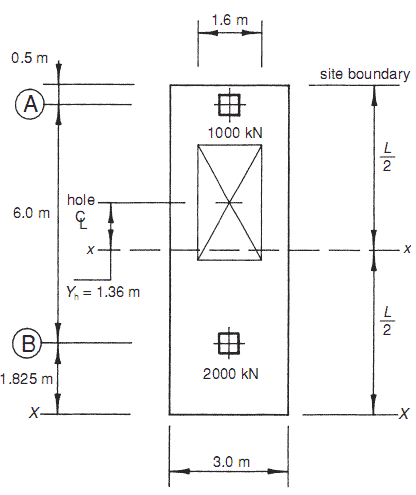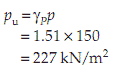## Thursday, February 7, 2013

### Design Example: Holed balanced foundation.

This example again makes use of the same building as in the previous examples. The trapezoidal shape will be squared off to give a 3.0 m × 8.325 m rectangular outline, as shown in Fig. 12.12.

To minimize differential settlements – both within the base and between adjacent bases – the combined base will be designed as a balanced foundation, with a bearing pressure equal to the allowable bearing pressure na = 150 kN/m2.

A balanced holed  foundation will be investigated for this example. By inserting a hole off-centre to the centroid of  the 3 m × 8.325 m base, it is possible to cause the centre  of gravity of the base to shift until it coincides with that of the applied loads.Fig. 12.12 Holed balanced foundation design example – loads.

The superstructure total load coming onto the base is given by

Area of hole
The area of the holed base is given by

A = (area of unholed base) − (area of hole)
= Au − Ah

From Fig. 12.12, the area of the unholed base is given by

Au = 3.0 × 8.325
= 24.5 m2

The size of the hole would optimally be chosen to give a bearing pressure equal to the allowable bearing pressure, i.e.

Thus the required area of the hole is

Ah = Au − A
= 25.0 − 20.0
= 5.0 m2

Condition for a balanced holed foundation
For a balanced foundation, the centre of gravity of the holed base is required to coincide with the centroid of applied loads. Let Y be the distance from the centre of the unholed base to the centroid of applied loads, and Yh the distance to the centre of the hole. From Figs 12.12 and 12.13, taking moments of area about x–x, Y is given by

YA = 0(Au) + Yh(Ah)

Thus

This is the condition for a balanced foundation.

From Fig. 12.12, Y = 0.34 m. ThusFig. 12.13 Holed balanced foundation design example – hole size.

Dimensions of base
To achieve a balanced foundation, the centre of the hole must be at a distance of Yh = 1.36 m from the centre of the unholed  base. Provided this condition is met, the actual shape of the hole, e.g. square or rectangular, is not critical.

The area of the hole was calculated earlier in this example as Ah = 5.0 m2. A rectangular hole will be adopted in this instance, having dimensions of 1.6 m × 3.125 m = 5.0 m2 (see Fig. 12.13).

Ultimate design pressure
The holed base has been sized to give a bearing pressure at working loads of 150 kN/m2 . The ultimate design pressure for reinforcement design, pu, is calculated as follows, with γP = 1.51 as in the previous example,